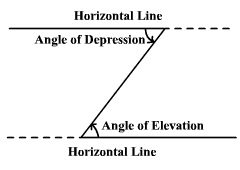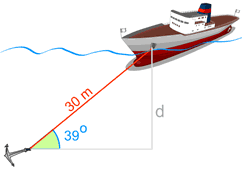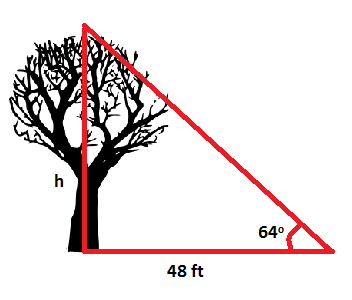Introduction:
The word “trigonometry” means “measurement of triangles”. Trigonometry has multiple applications to real world problems, it can be used in navigation, building, and engineering. But first, we need to understand the principles of trigonometry and how they are formed.

The Six Trigonometric Functions:
Trigonometric functions are defined by six functions. These functions apply to acute angles inside of triangles. The outputs of these functions are ratios that apply to the given triangle. For example in the triangle below the sin of angle A is the opposite side divided by the hypotenuse. The same concept applies to the other two functions. There are also 3 other trigonometric function that have a close relationship to the first 3 functions.SOHCAHTOA:
This is a helpful mnemonic device that can help you to remember the different relationships in the trigonometric functions. For example, Sin of an angle is opposite over hypotenuse, Cos of an angle is adjacent over the hypotenuse, and Tan of an angle is opposite over the adjacent side. The other 3 trig functions can be found by flipping the equation. So, Csc of an angle is hypotenuse over the opposite side from that angle, Sec of an angle is hypotenuse of the adjacent side from that angle, and Cot of an angle is the adjacent side of the angle over the opposite side from that angle.Practice Problems:
1.) Find the 6 trig functions of angle C:sinC°=__ cscC°=__

cosC°=__ secC°=__

tanC°=__ cotC°=__

2.) Find the 6 trig functions of angle C:sinC°=__ cscC°=__

cosC°=__ secC°=__

tanC°=__ cotC°=__

Answer:
1.)
sinC°= (2√6)/7 cscC°= (7√6)/12
cosC°= 5/7 secC°= 7/5
tanC°= (2√6)/5 cotC°= (5√6)/12

2.)
sinC°= ⅗ cscC°= 5/3
cosC°= ⅘ secC°= 5/4
tanC°= ¾ cotC°=4/3

*= Remember that square roots cannot be in the denominator for the trig functions

Rationalizing denominators:
Since square roots are not allowed in the denominator, here is the way around it.
Step one: If the radical in the denominator is a square root, then you multiply by a square root that will give you a perfect square under the radical when multiplied by the denominator.
Step two: Make sure all roots are simplified. If they are not simplified, refer to a previous section on how to simplify radicals.
Step three: Make sure the fraction is simplified
Examples 1:
cosC=24/√7
√7(24)/√7(√7)=24√7/7Inverse Functions:
The inverse functions of sin cos and tan are all used to “reverse” the functions. This is especially useful when evaluating for a side or angle of a triangle. The figure below should help you to understand the inverse sine, cosine, and tangent functions.Angle of Elevation/ Depression:
Looking up or down from a certain area creates an angle of elevation or depression. When you look up towards an airplane in the sky the angle of elevation could be 47°. Alternatively when looking down from a building to see someone on the street, the angle of depression could be 21°. A straight horizontal line is drawn from where you are standing which decides whether the angle is an elevation or depression. Above the line, it’s an elevation, below the line it’s a depression. For example the figure below shows the distinction between the two.How to Evaluate Angle of Elevation/ Depression Problems:
In problems like these to begin, a right triangle can be drawn using the angle of elevation/ depression. From here, it is easy to use right angle trig to solve for the unknown variable. The right angle can be formed using the horizontal line from the observer or any other plane that is perpendicular to the observer. For example, the figure below shows an example of an angle of elevation problem. Once the trig ratio has been found, you must use your knowledge of inverse functions to get the variable by itself and ultimately solve for the given variable.Practice Problems:

1.) Find side length d:2.) Find side length h:Answers:
1.) tan64°= h/48
tan64°x 48= h
h= 98.41 ft

2.) cos39°=d/30
cos39°x 30= d
d= 23.31 m